# Parallel and Perpendicular Assignment Parallel Lines Parallel lines

• Slides: 25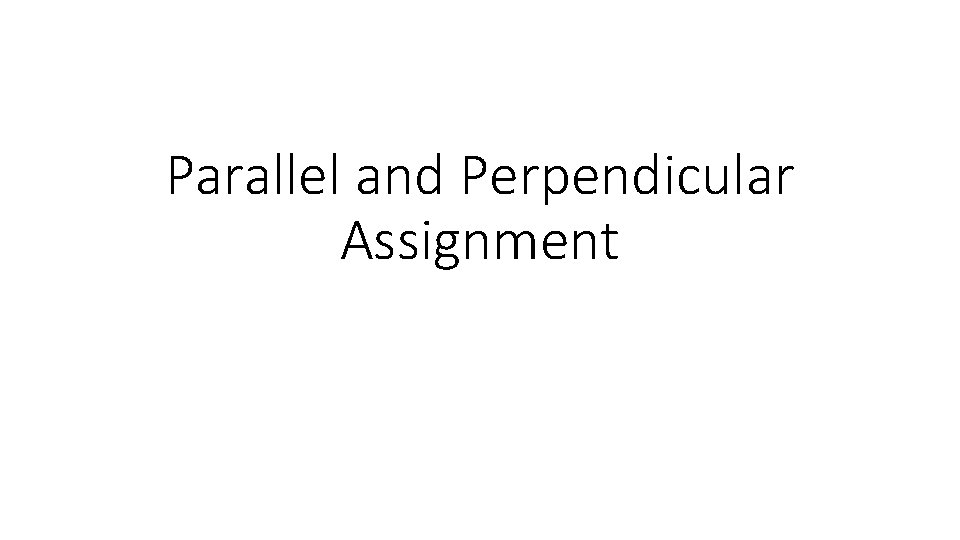Parallel and Perpendicular Assignment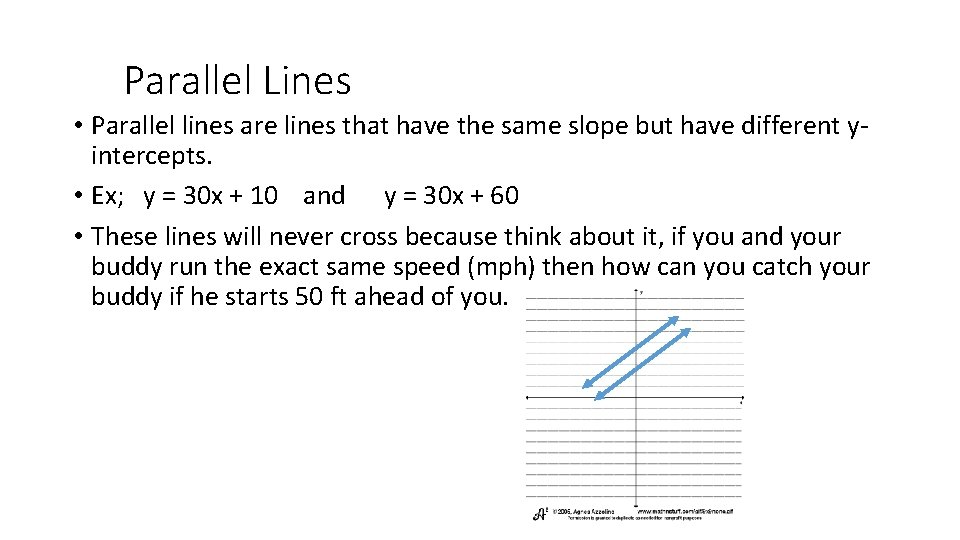Parallel Lines • Parallel lines are lines that have the same slope but have different yintercepts. • Ex; y = 30 x + 10 and y = 30 x + 60 • These lines will never cross because think about it, if you and your buddy run the exact same speed (mph) then how can you catch your buddy if he starts 50 ft ahead of you.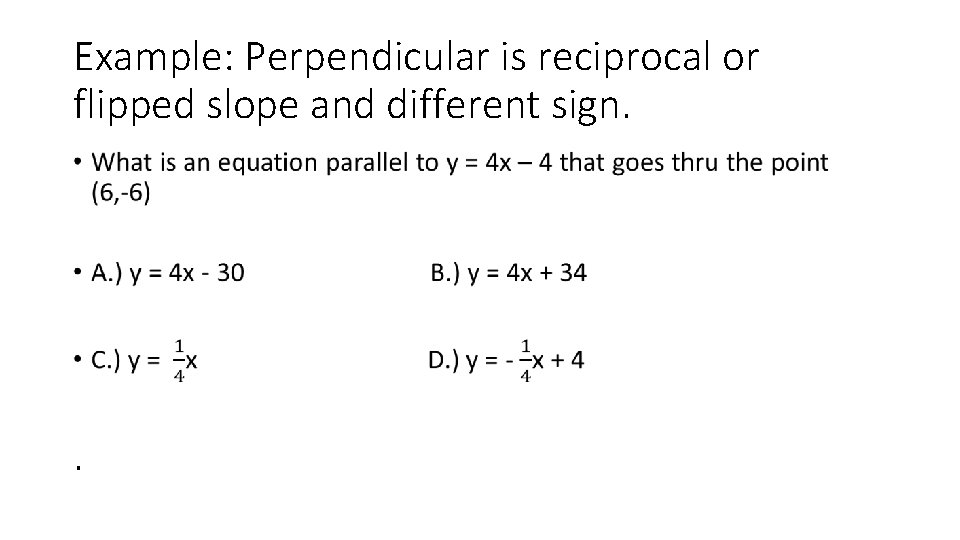Example: Perpendicular is reciprocal or flipped slope and different sign. • .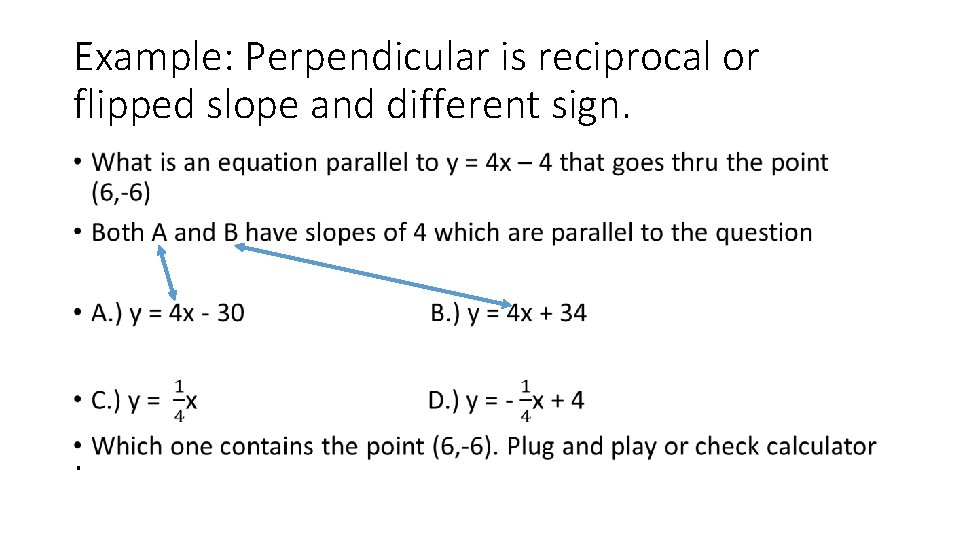Example: Perpendicular is reciprocal or flipped slope and different sign. • .Example: Perpendicular is reciprocal or flipped slope and different sign. • .Perpendicular lines •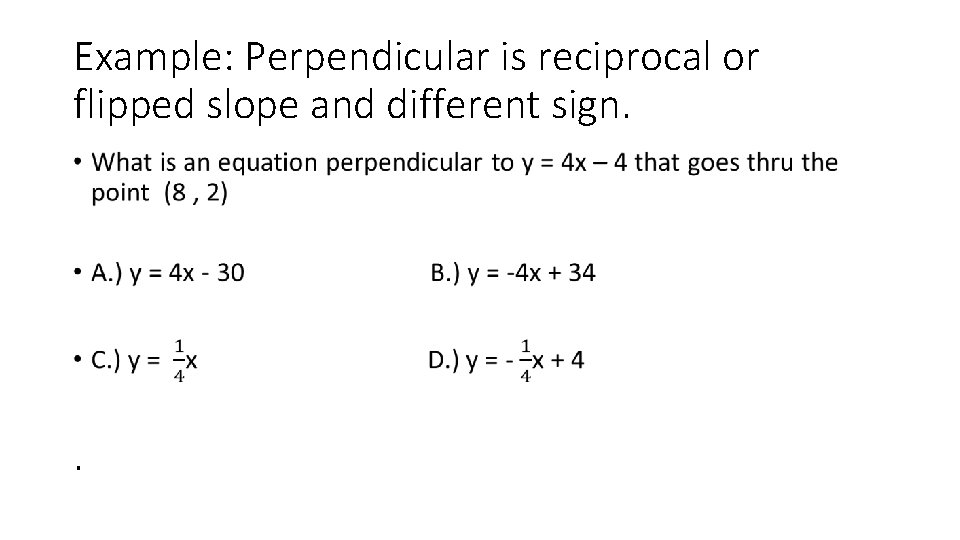Example: Perpendicular is reciprocal or flipped slope and different sign. • .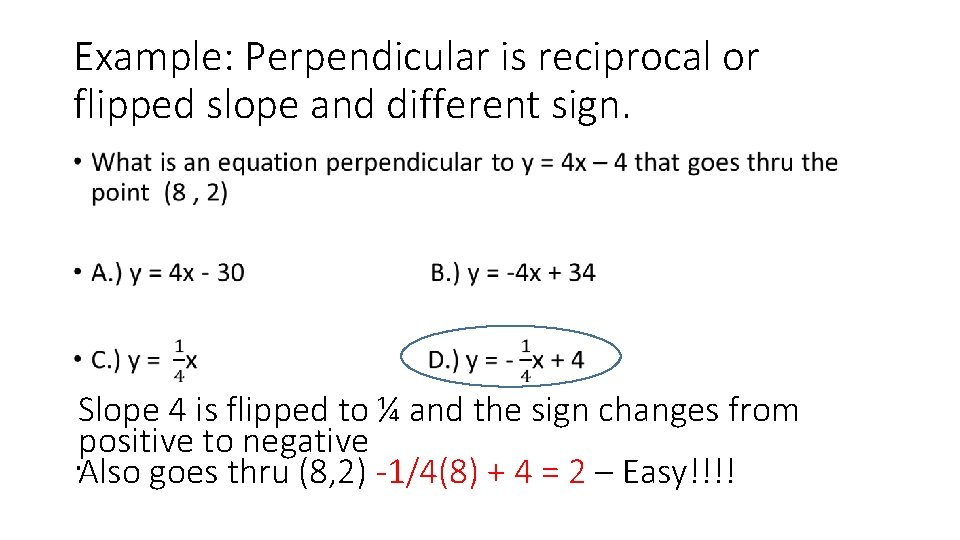Example: Perpendicular is reciprocal or flipped slope and different sign. • Slope 4 is flipped to ¼ and the sign changes from positive to negative. Also goes thru (8, 2) -1/4(8) + 4 = 2 – Easy!!!!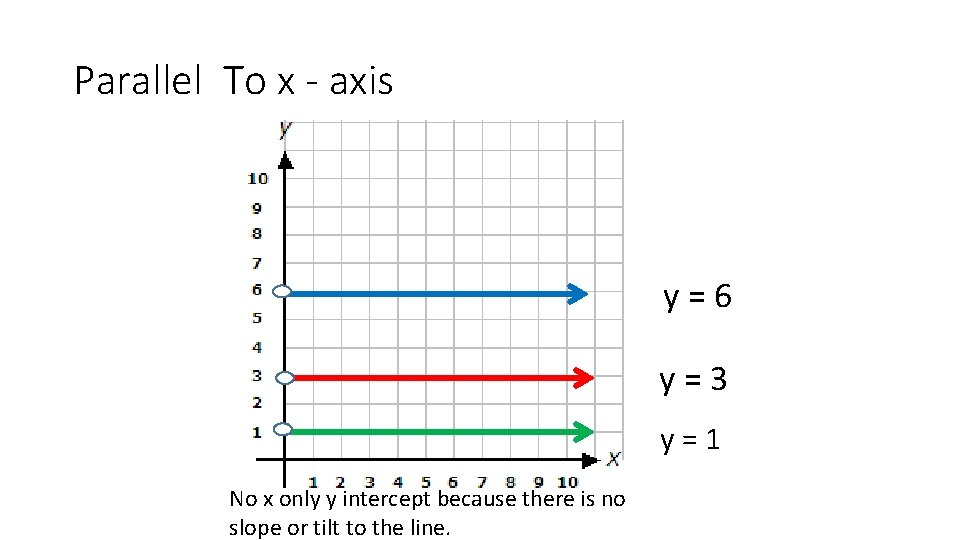Parallel To x - axis y = 6 y = 3 No x only y intercept because there is no slope or tilt to the line. y = 1Parallel To y - axis x = 2 x = 5 y = 9 No y = because the slope is undefined and there is no y intercepts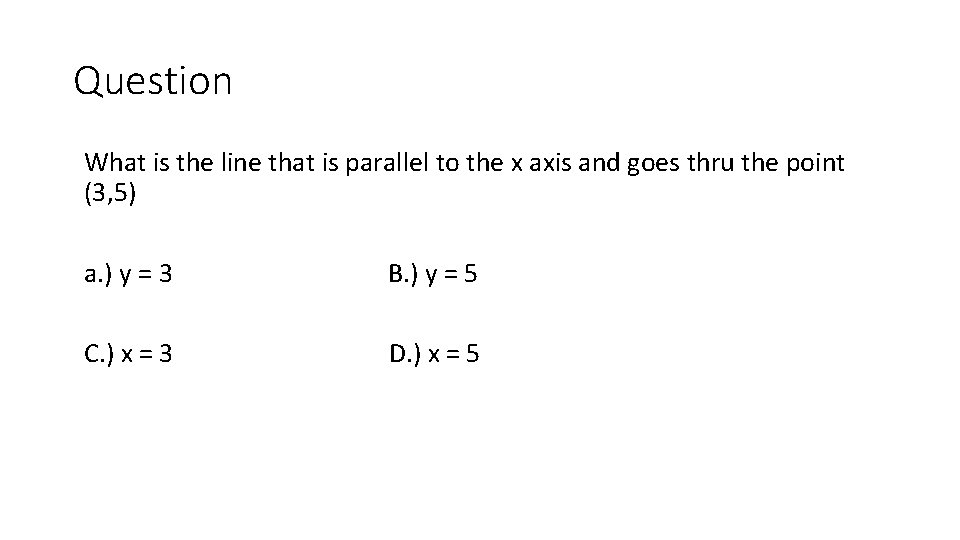Question What is the line that is parallel to the x axis and goes thru the point (3, 5) a. ) y = 3 B. ) y = 5 C. ) x = 3 D. ) x = 5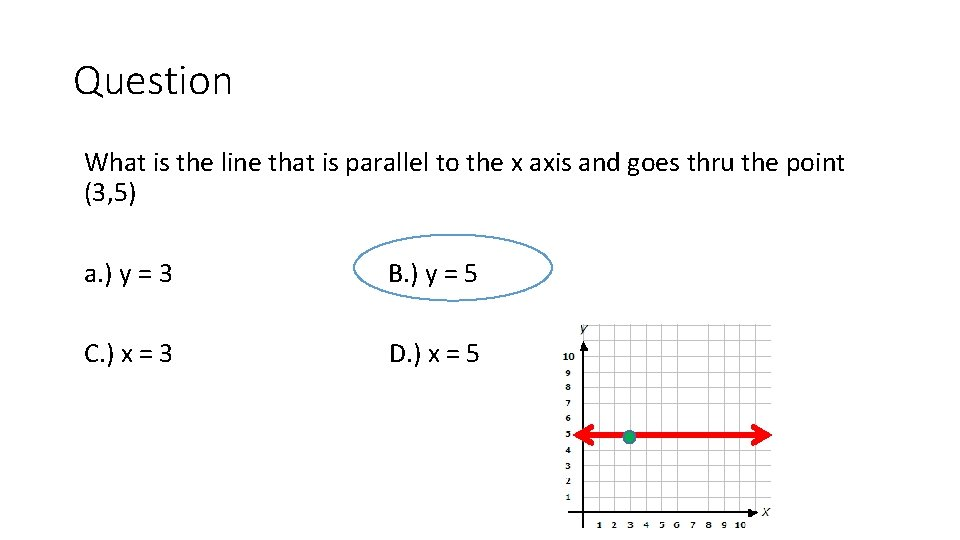Question What is the line that is parallel to the x axis and goes thru the point (3, 5) a. ) y = 3 B. ) y = 5 C. ) x = 3 D. ) x = 5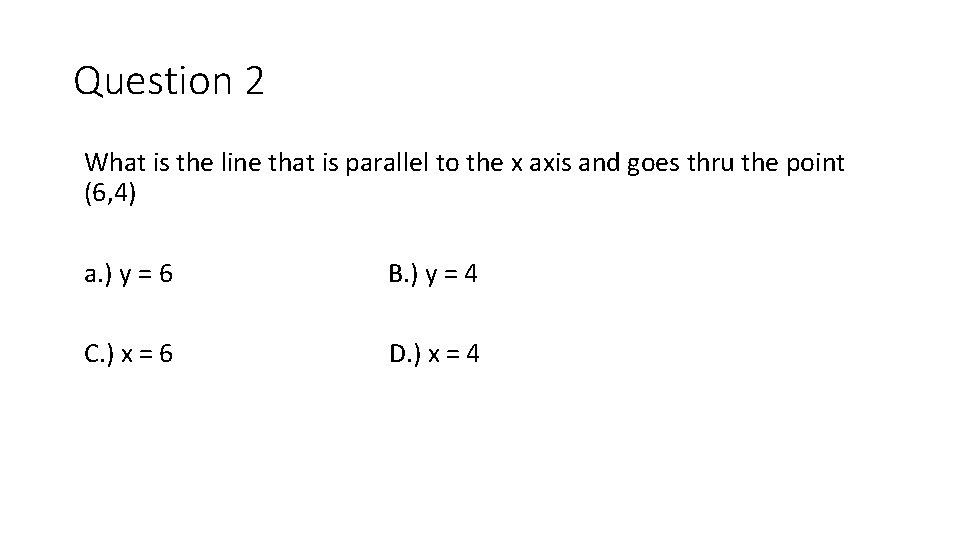Question 2 What is the line that is parallel to the x axis and goes thru the point (6, 4) a. ) y = 6 B. ) y = 4 C. ) x = 6 D. ) x = 4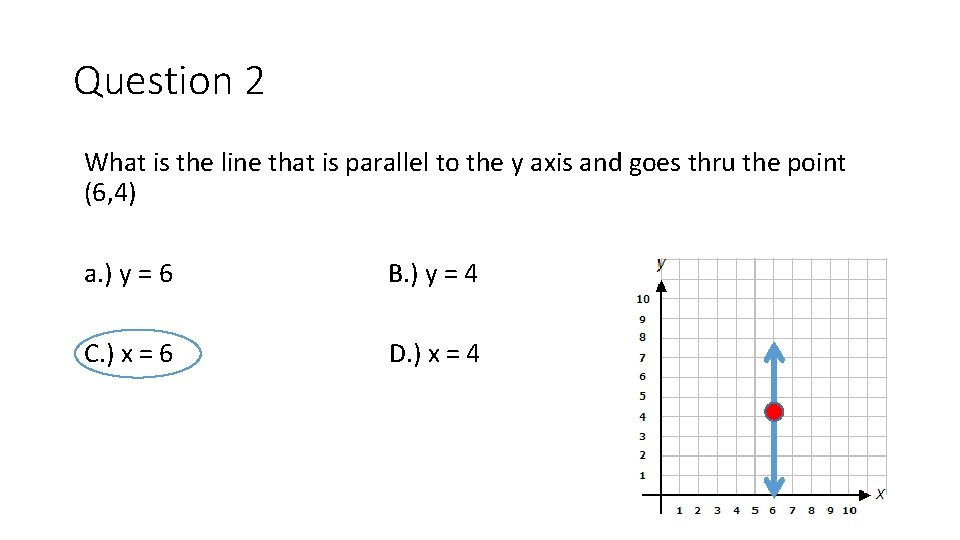Question 2 What is the line that is parallel to the y axis and goes thru the point (6, 4) a. ) y = 6 B. ) y = 4 C. ) x = 6 D. ) x = 4Assignment Question #1 •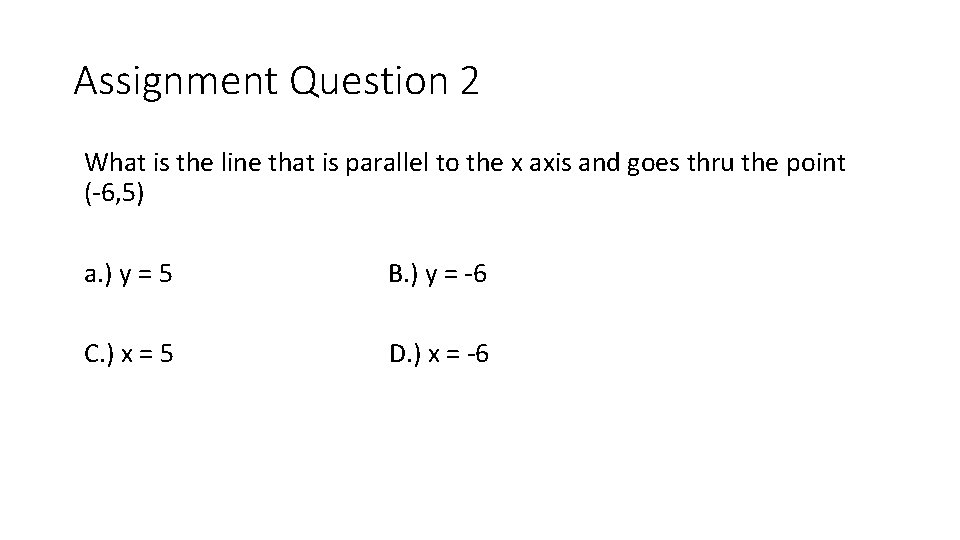Assignment Question 2 What is the line that is parallel to the x axis and goes thru the point (-6, 5) a. ) y = 5 B. ) y = -6 C. ) x = 5 D. ) x = -6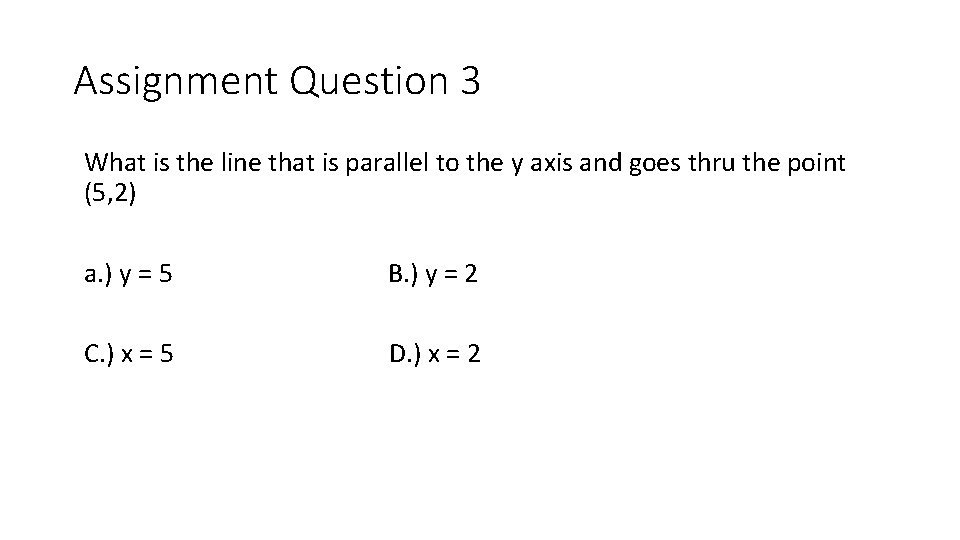Assignment Question 3 What is the line that is parallel to the y axis and goes thru the point (5, 2) a. ) y = 5 B. ) y = 2 C. ) x = 5 D. ) x = 2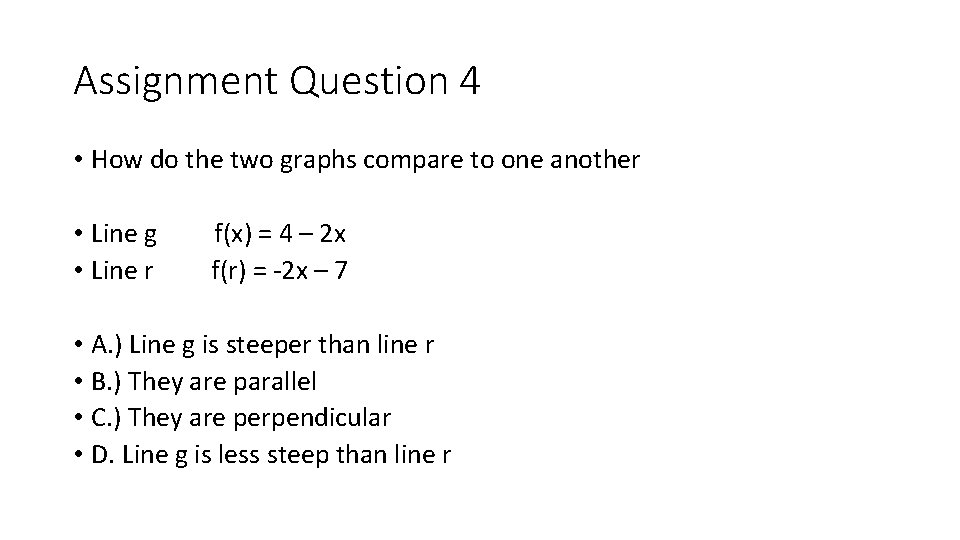Assignment Question 4 • How do the two graphs compare to one another • Line g f(x) = 4 – 2 x • Line r f(r) = -2 x – 7 • A. ) Line g is steeper than line r • B. ) They are parallel • C. ) They are perpendicular • D. Line g is less steep than line r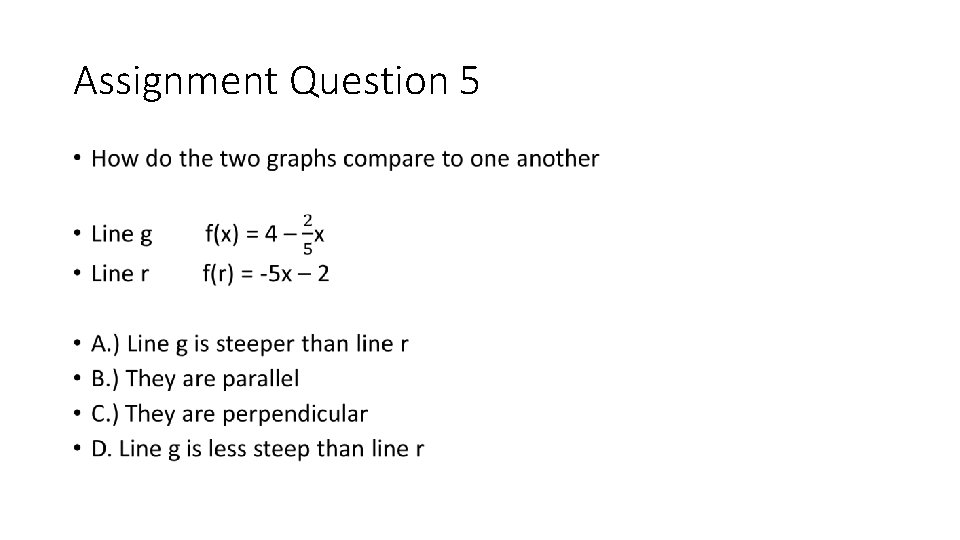Assignment Question 5 •Assignment Question 6 •Assignment Question 7Assignment Question 8Assignment Question 9Assignment Question #10 •Assignment Question 11 What is the line that is parallel to the y axis and goes thru the point (6, 3) a. ) 6 x + 3 y = 12 B. ) y = 3 C. ) x = 6 D. ) x = 3